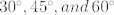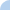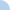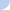# Trigonometric Ratios

This quiz covers reciprocals and cofunctions, as well as solving right triangles. You may use a calulator to find the values of trig ratios if angles are given in decimal degrees or DMS form. However, for special angles (such as) and when the values are given as fractions, you have to work with the exact values of trig ratios.

 This quiz is timed. The total time allowed for this quiz is 1 hour.Quiz Log In